Test: Measures of Central Tendency - 2

# Test: Measures of Central Tendency - 2 - SSC CGL

Test Description

## 10 Questions MCQ Test Statistics for SSC CGL - Test: Measures of Central Tendency - 2

Test: Measures of Central Tendency - 2 for SSC CGL 2023 is part of Statistics for SSC CGL preparation. The Test: Measures of Central Tendency - 2 questions and answers have been prepared according to the SSC CGL exam syllabus.The Test: Measures of Central Tendency - 2 MCQs are made for SSC CGL 2023 Exam. Find important definitions, questions, notes, meanings, examples, exercises, MCQs and online tests for Test: Measures of Central Tendency - 2 below.
Solutions of Test: Measures of Central Tendency - 2 questions in English are available as part of our Statistics for SSC CGL for SSC CGL & Test: Measures of Central Tendency - 2 solutions in Hindi for Statistics for SSC CGL course. Download more important topics, notes, lectures and mock test series for SSC CGL Exam by signing up for free. Attempt Test: Measures of Central Tendency - 2 | 10 questions in 10 minutes | Mock test for SSC CGL preparation | Free important questions MCQ to study Statistics for SSC CGL for SSC CGL Exam | Download free PDF with solutions
 1 Crore+ students have signed up on EduRev. Have you?
Test: Measures of Central Tendency - 2 - Question 1

### Find the mode of given samples. 47, 25, 15, 89, 47, 89, 89, 1, 47, 73, 29, 64, 95, 90, 47, 25.

Detailed Solution for Test: Measures of Central Tendency - 2 - Question 1

89 and 25 occurs twice, 64 occurs once, 47 occurs four times.
Since 47 has occurred four times in the above data set and other numbers less than that. The mode of the above data set is 47.

Test: Measures of Central Tendency - 2 - Question 2

### Find the median of 4.1, 5.2, 6.3, 3.6, 2.4, 6.4, 4.6

Detailed Solution for Test: Measures of Central Tendency - 2 - Question 2

Concept:
Median: It is the middle value in the given set, while the set is either in increasing or decreasing order.

If the number of observations (n) is odd then the median is (n+1)/2nd term.

If the number of observations (n) is even then the median is the average of nth and (n+1)th term.

Calculation:

Given data are 4.1, 5.2, 6.3, 3.6, 2.4, 6.4, 4.6

Increasing order of given data: 2.4, 3.6, 4.1, 4.6, 5.2, 6.3, 6.4

The number of observations is odd (n = 7)

So, the median is the  (n+1)/2th term or 4th term

Median is 4th term i.e., 4.6

Test: Measures of Central Tendency - 2 - Question 3

### A data set of n observations has mean 2M, while another data set of 2n observations has mean M. What is the mean of the combined data sets?

Detailed Solution for Test: Measures of Central Tendency - 2 - Question 3

Concept:

Combined Mean:

Let x1 and x2 are the means of the first and second group of data containing n1 and n2 items respectively.

Then combined mean is: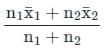Calculation:

Given:

n1 = n and x1 = 2M

n2 = 2n and x2 = M

The combined mean will be: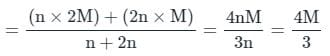Test: Measures of Central Tendency - 2 - Question 4

What is the mode of 19, 18, 9, 24, 1, 12, 23, 75?

Detailed Solution for Test: Measures of Central Tendency - 2 - Question 4

According to the definition of mode, a mode is a number that occurs most frequently. There is no such number which is repeated more than once . So, we can say there is No Mode.

Test: Measures of Central Tendency - 2 - Question 5

If mean and mode of some data are 4 & 10 respectively, its median will be:

Detailed Solution for Test: Measures of Central Tendency - 2 - Question 5

Given that,

mean of data = 4 and mode of  data = 10

We know that

Mode = 3(Median) - 2(Mean)

⇒ 10 = 3(median) - 2(4)

⇒ 3(median) = 18

⇒ median = 6

Hence, the median of data will be 6.

Test: Measures of Central Tendency - 2 - Question 6

Find the mean of given data: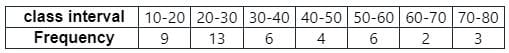Detailed Solution for Test: Measures of Central Tendency - 2 - Question 6

The mean of grouped data is given by,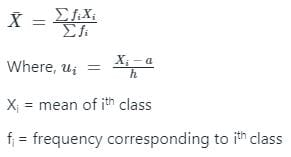Given: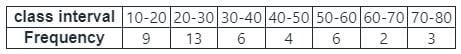Calculation:

Now, to calculate the mean of data will have to find ∑fiXi and ∑fi as below,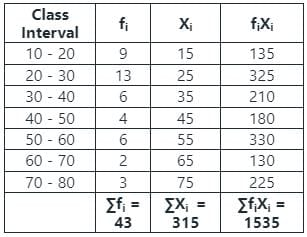Then,

We know that, mean of grouped data is given by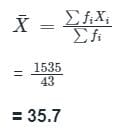Hence, the mean of the grouped data is 35.7

Test: Measures of Central Tendency - 2 - Question 7

What is the mode of 20, 15, 13, 12, 9, 17, 13, 1, 9?

Detailed Solution for Test: Measures of Central Tendency - 2 - Question 7

In the above data set, both 13 and 9 are repeated twice and a data set can have more than one mode. So, the mode is both 13 and 9.

Test: Measures of Central Tendency - 2 - Question 8

The Values of mean and median are given as 22.5 and 20 respectively. Find the approximate value of Mode.

Detailed Solution for Test: Measures of Central Tendency - 2 - Question 8

The empirical mean median mode relation is given as
Mean – Mode = 3(Mean – Median)
Given Mean = 22.5 and Median = 20
22.5 – Mode = 3(22.5 – 20)
Mode = 15

Test: Measures of Central Tendency - 2 - Question 9

Find the median of the given set of numbers 2, 6, 6, 8, 4, 2, 7, 9

Detailed Solution for Test: Measures of Central Tendency - 2 - Question 9

Concept:

Median: The median is the middle number in a sorted- ascending or descending list of numbers.

Case 1: If the number of observations (n) is even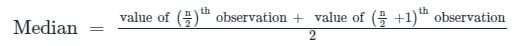Case 2: If the number of observations (n) is odd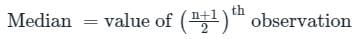Calculation:

Given values 2, 6, 6, 8, 4, 2, 7, 9

Arrange the observations in ascending order:

2, 2, 4, 6, 6, 7, 8, 9

Here, n = 8 = even

As we know, If n is even then,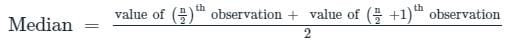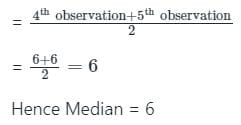Test: Measures of Central Tendency - 2 - Question 10

Find the mean of given data: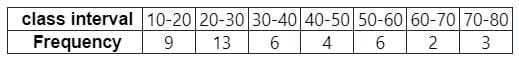Detailed Solution for Test: Measures of Central Tendency - 2 - Question 10

Formula used:

The mean of grouped data is given by,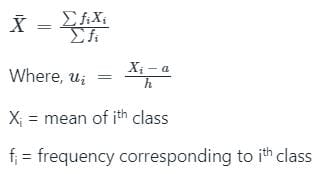Given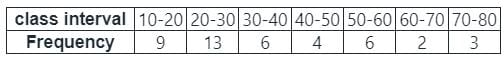Calculation:

Now, to calculate the mean of data will have to find ∑fiXi and ∑fi as below,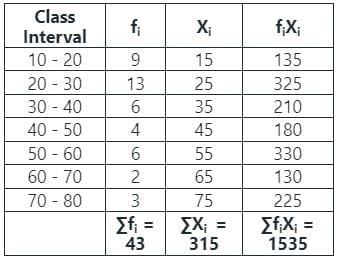Then,

We know that, mean of grouped data is given by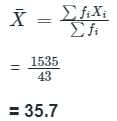Hence, the mean of the grouped data is 35.7

## Statistics for SSC CGL

69 videos|90 docs|16 tests
Information about Test: Measures of Central Tendency - 2 Page
In this test you can find the Exam questions for Test: Measures of Central Tendency - 2 solved & explained in the simplest way possible. Besides giving Questions and answers for Test: Measures of Central Tendency - 2, EduRev gives you an ample number of Online tests for practice

## Statistics for SSC CGL

69 videos|90 docs|16 tests